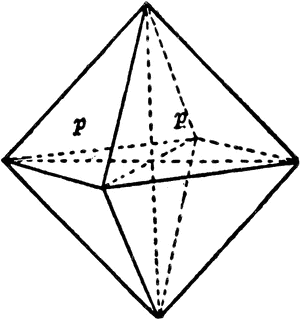# Find a distance in octahedron (simple stereometry)

This is quite simple question for this forum ;)

How to find distance in octahedron between centers of two adjacent faces, if its edge equals a?

First I assume you mean distance along the surface:

From the center of the triangle to the edge is half the sought distance $d$.

From the center $A$ of the triangle to the midpoint $B$ of an edge is the distance sought.

Let $C$ be one endpoint of that edge.

Then $\angle ABC=90^\circ$, $\angle BCA=30^\circ$, and $\angle CAB = 60^\circ$.

If the distance from $A$ to $B$ is $d/2$, then the length of the hypotenuse $AC$ is $d$ and the length of the longer leg $BC$ is $d\sqrt{3}/2$. So $d\sqrt{3}= a/2$. Therefore $$d= \frac{a}{2\sqrt{3}} = \frac{a\sqrt{3}}{6}.$$Next I assume you mean distance through the interior. This one is simpler. Let the coordinates of three adjacent vertices be $(1,0,0)$, $(0,1,0)$, and $(0,0,1)$. Then the center of that face is the average of those three: $(1/3,1/3,1/3)$.

The vertices of an adjoining face are $(1,0,0)$, $(0,1,0)$, and $(0,0,-1)$. The center of that face is the average: $(1/3,1/3,-1/3)$.

The distance between those two centers is the norm of the difference, i.e. the norm of $(1/3,1/3,1/3)-(1/3,1/3,-1/3)=(0,0,2/3)$. That is $\sqrt{0^2+0^2+(2/3)^2}=2/3$.

The distance as measured through the interior of the octahedron is easily found by placing the vertices on a coordinate system, then scaling as necessary. If $(1,0,0), (0,1,0), (0,0,1)$ are three vertices of a single face, then the center of that face is $(1/3, 1/3, 1/3)$. Similarly, the center of an adjacent face is $(1/3, -1/3, 1/3)$, and the distance between the centers is simply $2/3$. The edge length of such an octahedron is obviously $\sqrt{2}$, hence if we scale accordingly to an octahedral edge length of $a$, then the distance between adjacent centers is simply $\frac{2}{3 \sqrt{2}} a$.